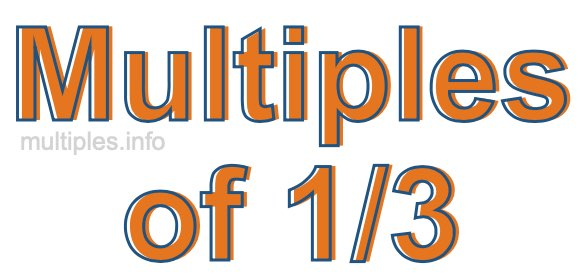Multiples of 1/3We define the multiples of 1/3 as the product of any integer multiplied by 1/3. Therefore, to create a list of multiples of 1/3 we start by multiplying 1/3 by one, then we multiply 1/3 by two, then we multiply 1/3 by three, and so on.

To multiply a fraction by an integer, we leave the denominator alone and multiply the numerator by the integer. It is impossible to give you a list of all multiples of 1/3 because the list goes on forever. However, here is the math to calculate the beginning list of multiples of 1/3.

1/3 × 1 = 1/3

1/3 × 2 = 2/3

1/3 × 4 = 3/3

1/3 × 4 = 4/3

1/3 × 5 = 5/3

1/3 × 6 = 6/3

1/3 × 7 = 7/3

1/3 × 8 = 8/3

1/3 × 9 = 9/3

1/3 × 10 = 10/3

1/3 × 11 = 11/3

1/3 × 12 = 12/3

We have simplified the fractions above for you. Below are the multiples of 1/3 in their lowest possible terms:

1/3 = 1/3

2/3 = 2/3

3/3 = 1

4/3 = 1 1/3

5/3 = 1 2/3

6/3 = 2

7/3 = 2 1/3

8/3 = 2 2/3

9/3 = 3

10/3 = 3 1/3

11/3 = 3 2/3

12/3 = 4

As we stated above, the list of multiples of 1/3 goes on forever because the product of 1/3 multiplied by any whole number (integer) is a multiple of 1/3. You can also create a list of multiples of 1/3 if you keep adding 1/3 like this:

1/3
1/3 + 1/3 = 2/3
1/3 + 1/3 + 1/3 = 3/3
1/3 + 1/3 + 1/3 + 1/3 = 4/3

Again, the list can go on forever, because you can continue adding 1/3 to the list. Therefore, the list of multiples of 1/3 is ∞ (infinite).

Multiples of Fractions
Do you need the multiples of another fraction? Enter a another fraction below to get the multiples of that fraction.

/

Multiples of 1/4
Go here for the next fraction on our list that we have multiples for.